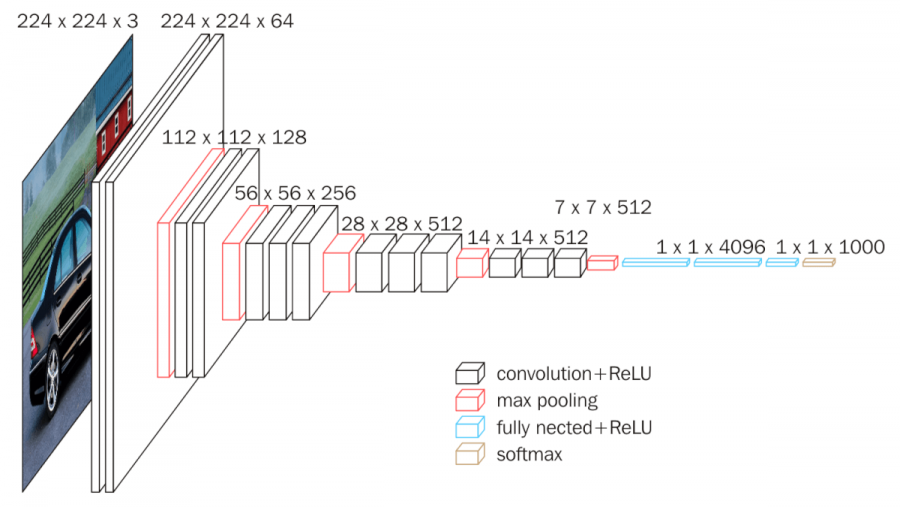# Facial KeyPoint Detection with Pytorch

Source: Deep Learning on Medium# Facial KeyPoint Detection with Pytorch

## Introduction

Time is passing and I am still quite passionate about Deep Learning. For this reason, I have decided to dig deeper into the sub-field of Computer Vision, CV hereinafter, which I think will redefine human life in the next few decades. If machines can see, there is a lot of things they are going to be able to do for us. Some philosophical concerns here too, or a lot of them rather, but that is for another post.

Following my increasing interest in medicine, I have decided to implement a facial keypoint detector, that runs on a fairly straightforward convolutional neural network, CNN hereinafter. Software of this sort will allow us to catch disease earlier. I have used Pytorch to build this.

Before the sense of overwhelm kicks in (happens to me a lot), here´s a quick step by step summary of the project:

1. Get data (pictures of faces with corresponding keypoint coordinates).
2. Apply transformations on it, to help the network learn.
3. Define the network.
4. Train the network and test it.
5. Use the network to make predictions (in this case, return facial keypoints).

## 1 – Get data

I am using this dataset. Each data point is an image of a face with its corresponding 68 keypoints. Each keypoint is an (x,y) coordinate. It looks like this:

If you have a little bit of Deep Learning experience, you will already know where this is heading. The image (without the keypoints) is a 3 dimensional array of pixels. The keypoints are simply 136 numbers (68 coordinate pairs). We are basically going to teach the network to correctly predict 136 numbers.

Store the data in a given directory, such as :

‘/data/training_frames_keypoints.csv’

Any given data point looks like this:

`Image name: Luis_Fonsi_21.jpgLandmarks shape: (68, 2)First 4 key pts: [[ 45. 98.] [ 47. 106.] [ 49. 110.] [ 53. 119.]]`

## 2— Transform the data

Transforming data adequately helps a neural network learn. For CV, it is almost always about:

1. Reducing the initial dimensionality of the image , transforming image from RGB (3 2D arrays) to greyscale (1 2D arrays).
2. Rescaling and random cropping it.
3. Normalising pixel values.
4. Finally transforming the image into a tensor data type.

In this project, I have dealt with this section in an object oriented way, initially defining a class for the dataset, that actually inherits from the Dataset class from torch.utils.data. This allows to work on data in a more agile fashion down the line.

`from torch.utils.data import Dataset, DataLoaderclass FacialKeypointsDataset(Dataset): """Face Landmarks dataset."""def __init__(self, csv_file, root_dir, transform=None): """ Args: csv_file (string): Path to the csv file with annotations. root_dir (string): Directory with all the images. transform (callable, optional): Optional transform to be applied on a sample. """ self.key_pts_frame = pd.read_csv(csv_file) self.root_dir = root_dir self.transform = transformdef __len__(self): return len(self.key_pts_frame)def __getitem__(self, idx): image_name = os.path.join(self.root_dir, self.key_pts_frame.iloc[idx, 0]) image = mpimg.imread(image_name) # if image has an alpha color channel, get rid of it if(image.shape == 4): image = image[:,:,0:3] key_pts = self.key_pts_frame.iloc[idx, 1:].as_matrix() key_pts = key_pts.astype('float').reshape(-1, 2) sample = {'image': image, 'keypoints': key_pts}if self.transform: sample = self.transform(sample)return sample`

This allows for a dataset to be created quite quickly, as follows:

`#creating dataset, by instantiating FacialKeyPointsDataset classface_dataset = FacialKeyPointsDataset(csv_file='/data/training_frames_keypoints.csv',root_dir='/data/training/')`